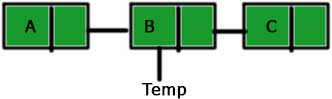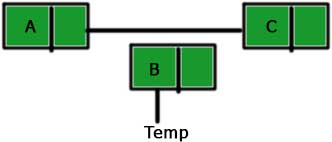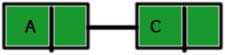# Deletion of a given node from a linked list in C

May 25, 2017 126477

### Previous:

Make sure that you are familiar with the concepts explained in the article(s) mentioned above before proceeding further.

We will proceed further by taking the linked list we made in the previous article.

#include <stdio.h>
#include <stdlib.h>

struct node
{
int data;
struct node *next;
};

int main()
{
int n,i;
printf ("number of elements:");
scanf("%d",&n);
for(i=0;i<n;i++)
{
p=malloc(sizeof(struct node));
scanf("%d",&p->data);
p->next=NULL;
else
prev->next=p;
prev=p;
}
return 0;
}


We delete any node of a linked list by connecting the predecessor node of the node to be deleted by the successor node of the same node. For example, if we have a linked list a → b → c, then to delete the node ‘b’, we will connect ‘a’ to ‘c’ i.e., a → c. But this will make the node ‘b’ inaccessible and this type of inaccessible nodes are called garbage and we need to clean this garbage. We do this cleaning by the use of ‘free’ function. If you are not familiar with the ‘free’ function then you can visit the ‘Dynamic memory’ chapter of the C course. So, the steps to be followed for deletion of the node ‘B’ from the linked list A → B → C are as follows:

1. Create a temporary pointer to the node ‘B’.2. Connect node ‘A’ to ‘B’.3. Free the node ‘B’.The code representing the above steps is:

del (struct node *before_del)
{
struct node *temp;
temp = before_del->next;
before_del->next = temp->next;
free(temp);
}


Here, ‘before_node’ is the predecessor of the node to be deleted.
temp = before_del->next – We are making a temporary pointer to the node to be deleted.
before_del->next = temp->next – Connecting the predecessor of the node to be deleted with the successor of the node to be deleted.
free(temp) – Making the ‘temp’ free.

And the overall code is:

#include <stdio.h>
#include <stdlib.h>

struct node
{
int data;
struct node *next;
};

{
{
printf("NULL\n");
}
else
{
}
}

del (struct node *before_del)
{
struct node *temp;
temp = before_del->next;
before_del->next = temp->next;
free(temp);
}

int main()
{
int n,i;
printf ("number of elements:");
scanf("%d",&n);
for(i=0;i<n;i++)
{
p=malloc(sizeof(struct node));
scanf("%d",&p->data);
p->next=NULL;
else
prev->next=p;
prev=p;
}
/*node to be deleted is head->next->next*/